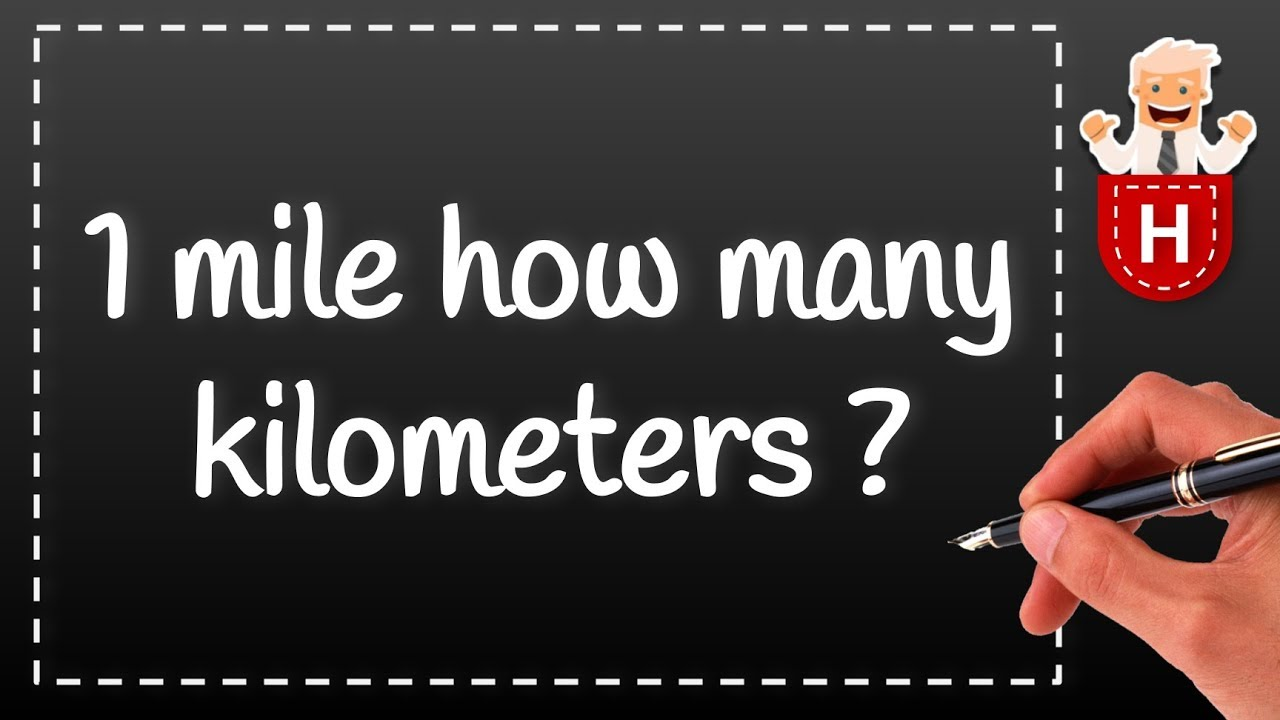News

# Conversion of Kilometers and MilesWe will be looking at how to convert kilometers to miles and how also to convert miles to kilometers.

### How Many Kilometer Make One Mile

The number of Kilometer that makes one mile is 1.60934 kilometers. That’s 1.609 kilometers make up one Mile.

For example, 1 mi=1.60934 km/1 mi or by

1 km=1 mi/0.62137 km

This will therefore cancel the miles out of it.

### How many Miles make one Kilometer

The number of Miles that will make one kilometer is 0.62137 miles. That is 0.621 miles make up one kilometer.

1 km=1 mi/1.60934 km or by

1 mi = 0.62137 km/1 mi

If we do this, the multiplication will cause the kilometers to cancel out.

1 mile = 1.6 km

1/10 mile = 0.16 km
1/8 mile = 0.2 km
1/4 mile = 0.4 km
1/2 mile = 0.8 km
1 mile = 1.6 km
1.5 mile = 2.4 km
2 miles = 3.2 km
2.5 miles = 4 km
5 miles = 8 km
10 miles = 16.1 km
15 miles = 24.1 km
20 miles = 32.2 km
25 miles = 40.2 km
50 miles = 80.4 km
75 miles = 120.7 km
100 miles = 160.9 km

Let’s look at one example below.

Convert 500 kilometers to miles.

500 km× 1 mi/1.60934 km

=500 km×1 mi/1.60934 km

=500 km×1 mi/1.60934 km

=310.686 mi

### Alex Peter Onoja

A passionate blogger who want more than ordinary from life

Check Also
Close